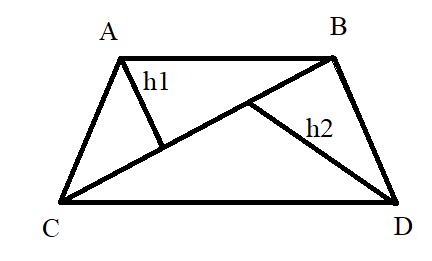Courses
Courses for Kids
Free study material
Offline Centres
MoreLast updated date: 24th Nov 2023
Total views: 279.6k
Views today: 2.79k

# Find the area of a quadrilateral whose diagonal length is $10cm$, height ${h_1} = 5cm$ and height ${h_2} = 7cm$ .Verified
279.6k+ views
Hint: In this problem we need to find area of quadrilateral, since they have given height of triangle as $5cm$ and $7cm$ we can find the area of triangle individually and add the area of triangle to get the area of quadrilateral.

Complete step-by-step solution:
Let us consider a quadrilateral $ABCD$ as shown below.
Let ${h_1}$ be the height of triangle $ABC$ and ${h_2}$ be the height of the triangle $BDC$Given:
$BC = 10cm$
${h_1} = 5cm$ and ${h_2} = 7cm$
We know that, area of quadrilateral = area of triangle $ABC$ $+$ area of triangle $BCD$
And also, area of triangle $= \dfrac{1}{2} \times base \times height$
Therefore, area of quadrilateral $= \dfrac{1}{2} \times BC \times {h_1} + \dfrac{1}{2} \times BC \times {h_2}$
Substituting the given data in above equation we get,
$= \left( {\dfrac{1}{2} \times 10 \times 5 + \dfrac{1}{2} \times 10 \times 7} \right)c{m^2}$
On simplifying,
$= \left( {25 + 35} \right)c{m^2}$
Therefore,
Area of quadrilateral $ABCD$ $= 60c{m^2}$.

Note: The quadrilateral is the combination of the basic geometric shapes which includes two triangles. Two calculate the area of a quadrilateral, the area of two individual triangles should be computed and the area of the individual triangle should be added. The quadrilateral is the closed, two dimensional figure which has four sides.